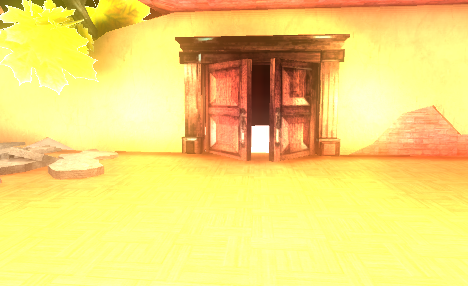# CharacterController.isGrounded not working after Unity 5

Since I upgraded to Unity 5 this script no longer works. I have confirmed the program will run but the If (CharacterController.isGrounded) loop won’t run. Does anybody know if this code no longer works? Thanks to anyone who could please tell me how to get this working.

I got the script from here

``````#pragma strict
// This script moves the character controller forward
// and sideways based on the arrow keys.
// It also jumps when pressing space.
// Make sure to attach a character controller to the same game object.
// It is recommended that you make only one call to Move or SimpleMove per frame.

var speed : float = 6.0;
var jumpSpeed : float = 8.0;//how high it jumps even though its called speed
var gravity : float = 20.0;
var rotateSpeed : float = 3.0;

private var moveDirection : Vector3 = Vector3.zero;//static open to whole project, private

function Update() {
var controller : CharacterController = GetComponent.<CharacterController>();
print("Line 17"); // DELETE
if (controller.isGrounded) {
print("Line 19"); // DELETE
// We are grounded, so recalculate
// move direction directly from axes
moveDirection = Vector3(0, 0, Input.GetAxis("Vertical"));

//rotate code
transform.Rotate(0, Input.GetAxis("Horizontal")* rotateSpeed, 0);

moveDirection = transform.TransformDirection(moveDirection);
moveDirection *= speed;

if (Input.GetButton ("Jump")) {
moveDirection.y = jumpSpeed;
}
}

// Apply gravity
moveDirection.y -= gravity * Time.deltaTime;

// Move the controller
controller.Move(moveDirection * Time.deltaTime);
}
``````

I had the same problem and my fix was to basically call:

controller.Move(moveDirection * Time.deltaTime);

BEFORE calling:

if (controller.isGrounded)

…some logic might have to be reorganized a bit but it was a pretty simple fix (although it took me days to figure out!). What’s strange is that the old (4.6) behavior of checking .isGrounded before calling controller.Move() still works with certain objects. Not sure why. I guess it doesn’t matter, just make sure to always call the Move() method first from now on and you should be fine.

You have to push the charactercontroller to the ground with the gravity, and check the isgrounded after the “move” function.

``````public float gravity = 10f;
public float maxFallSpeed = 10f;
CharacterController cc;
bool ground = false;

void Start()
{
cc = GetComponent<CharacterController>();​
}

void Update()
{
if(ground)
{
Y = Mathf.Clamp(Y, -0.1f, Mathf.Infinity);​
}
else
{
Y = Mathf.Clamp(Y - gravity * Time.deltaTime, -maxFallSpeed, Mathf.Infinity); //This is just a basic gravity, the point is to take the character down when it’s in the air, but don’t stop it from jumping.​

cc.Move(new Vector3(0, Y, 0) * Time.deltaTime); // insert here the motion values
ground = cc.isGrounded; //check the isgrounded after the "move" function, like this.​
}​
``````

I work in C# and i had this problem too. I did a port from unity 4 and it works perfectly, but there are some problems in unity 5 with “isGrounded”. I put the player 1 unit above the ground and is not working (is grounded works perfectly with mesh collider).
Here is when the player is colliding with the mesh collider (the mesh was made in blender)but the player passes through the ground when it collides with it.

Also, there is a problem that when i select a brush on “paint height”, i only can paint a circle

we have to wait until the developers solve it.

I’m having the same problem in Unity 5 in that my character won’t jump but the other input functions work properly with the following code (I got this from a tutorial):

``````using UnityEngine;
using System.Collections;

public class PlayerMovement : MonoBehaviour
{
CharacterController _controller;

[SerializeField]
float _moveSpeed = 5.0f;

[SerializeField]
float _jumpSpeed = 20.0f;

[SerializeField]
float _gravity = 1.0f;

float _yVelocity = 0.0f;

// Use this for initialization
void Start ()
{
_controller = GetComponent<CharacterController> ();
}

// Update is called once per frame
void Update ()
{

Vector3 direction = new Vector3 (Input.GetAxis ("Horizontal"), 0, Input.GetAxis ("Vertical"));
Vector3 velocity = direction * _moveSpeed;

if (_controller.isGrounded)
//print ("We are grounded");
{
if(Input.GetButtonDown("Jump"))
{
_yVelocity = _jumpSpeed;

}
else
{
_yVelocity -= _gravity;
}
}

velocity.y = _yVelocity;

_controller.Move (velocity * Time.deltaTime);

}
}
``````

However, if I un-comment print (“We are grounded”); the script works like it should and the character (capsule in this case) jumps. I’m a beginner at coding so I don’t really know what is happening and don’t understand why the print function would have that effect because from what I have been reading it shouldn’t. Is it a bug? lol.